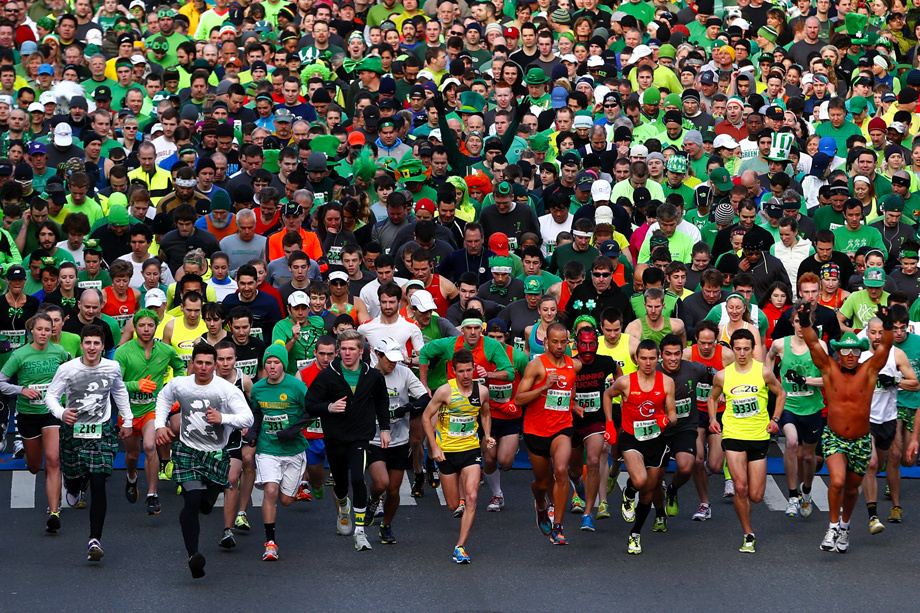# Percent kinematicsA runner completes a 12km race with an average velocity ($\overline { { v }_{ total } }$) of $4\text{ m/s}$. She does this in a certain amount of time, and after running for the first 16% of this time, her average velocity is $8\text{ m/s}$ when she reaches a checkpoint. Afterwards, she continues running for the same amount of time again. By the end of this time, she covers the first 50% of the race's distance.

Using the information given, calculate the runner's average velocity, $\overline { { v }_{ mid } }$, from the checkpoint up to this halfway point. Express your answer as the percent decrease from $\overline { { v }_{ mid } }$ to $\overline { { v }_{ total } }$.

Details and Assumptions

Do not calculate the average velocity as time-weighted; use the following formula: $\overline { v } =\frac { \Delta x }{ \Delta t } =\frac { { x }_{ f }-{ x }_{ i } }{ { t }_{ f }-{ t }_{ i } }$ Treat all acceleration as constant; there is no need for calculus.

×